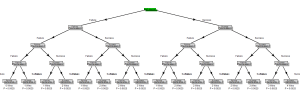# Diagram for a Bernoulli process (using R)

A Bernoulli process is a sequence of Bernoulli trials (the realization of n binary random variables), taking two values (0/1, Heads/Tails, Boy/Girl, etc…). It is often used in teaching introductory probability/statistics classes about the binomial distribution. When visualizing a Bernoulli process, it is common to use a binary tree diagram in order to show the […]

A Bernoulli process is a sequence of Bernoulli trials (the realization of n binary random variables), taking two values (0/1, Heads/Tails, Boy/Girl, etc…). It is often used in teaching introductory probability/statistics classes about the binomial distribution.

When visualizing a Bernoulli process, it is common to use a binary tree diagram in order to show the progression of the process, as well as the various consequences of the trial. We might also include the number of “successes”, and the probability for reaching a specific terminal node.

I wanted to be able to create such a diagram using R. For this purpose I composed some code which uses the {diagram} R package. The final function should allow one to create different sizes of diagrams, while allowing flexibility with regards to the text which is used in the tree.

Here is an example of the simplest use of the function:

```source("https://www.r-statistics.com/wp-content/uploads/2011/11/binary.tree_.for_.binomial.game_.r.txt") # loading the function
binary.tree.for.binomial.game(2) # creating a tree for B(2,0.5)
```

The resulting diagram will look like this:The same can be done for creating larger trees. For example, here is the code for a 4 stage Bernoulli process:

```source("https://www.r-statistics.com/wp-content/uploads/2011/11/binary.tree_.for_.binomial.game_.r.txt") # loading the function
binary.tree.for.binomial.game(4) # creating a tree for B(4,0.5)
```

The resulting diagram will look like this:The function can also be tweaked in order to describe a more specific story. For example, the following code describes a 3 stage Bernoulli process where an unfair coin is tossed 3 times (with probability of it giving heads being 0.8):

```source("https://www.r-statistics.com/wp-content/uploads/2011/11/binary.tree_.for_.binomial.game_.r.txt") # loading the function

binary.tree.for.binomial.game(3, 0.8, first_box_text = c("Tossing an unfair coin", "(3 times)"), left_branch_text = c("Failure", "Playing again"), right_branch_text = c("Success", "Playing again"),
left_leaf_text = c("Failure", "Game ends"), right_leaf_text = c("Success",
box_color = "lightgrey", shadow_color = "darkgrey", left_arrow_text = c("Tails n(P = 0.2)"),
right_arrow_text = c("Heads n(P = 0.8)"), distance_from_arrow = 0.04)
```

The resulting diagram is:If you make up neat examples of using the code (or happen to find a bug), or for any other reason – you are welcome to leave a comment.

(note: the images above are licensed under CC BY-SA)

Posted on Categories R, R bloggers

This site uses Akismet to reduce spam. Learn how your comment data is processed.